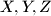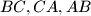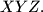IMO Shortlist 2010 problem G6

Kvaliteta:
Avg: 0,0
Težina:
Avg: 8,0
The vertices$X, Y , Z$ of an equilateral triangle$XYZ$ lie respectively on the sides$BC, CA, AB$ of an acute-angled triangle$ABC.$ Prove that the incenter of triangle$ABC$ lies inside triangle$XYZ.$

Proposed by Nikolay Beluhov, Bulgaria
Izvor: Međunarodna matematička olimpijada, shortlist 2010